## Gaussian Elimination

Gaussian elimination. It sounds terrible, and that's why most people run the other way when they see it coming. Not us. We're awesome like that. We are going to teach you that it's just a small extension of the elimination method in solving systems of equations (from Algebra 1 and Algebra 2), but with a few extra tricks called row operations.

Learning about Gaussian elimination will give you the capability of solving many variable (multivariate) systems. If you like a challenge, you are in for a treat. A Gaussian treat, that is.

We have been studying on the intersection of two lines in a 2-dimensional plane. Did you know that an equation that looks like this:

x + y + z = 3

...is not a line. It's a bird. It's superman. Okay, it's actually a plane. Think about 3D space. Look around right where you are sitting. Remember back to Geometry when we learned that three points determine a plane. Pick three spots in the space in front of your face. Each of those points in the space floating in front of your face have an x, y, and z coordinate. Now picture laying a piece of paper right on top of those three points. The equation above actually defining a plane in space.

Check out the plane that was defined in the 3D equation above: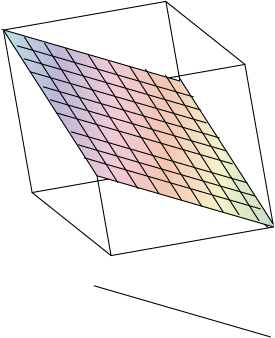Can you picture three planes intersecting in 3D space? Can you picture the edge of a cube where three planes come together? The intersection of three planes will usually be a point with an x,y,z coordinate that is in the format (x, y, z).

Sometimes there is no solution to three. This happens if there the planes are parallel planes. Sometimes there are infinite solutions if the three planes are the same plane.

Solving equations with more than two variables are called multi-variate systems of equations. Try to remember that all we are trying to find is the coordinate in which these equations intersect.

### Sample Problem

Solve this system of equations by the substitution method:

x – 4y + z = 14
6yz = -10
4z = -8

Notice that the first equation has x, y, and z, the second equation has y and z, and the last equation has z. This is triangular form and is very easy to solve for the intersection. Start at the bottom and work up! Solve for z, plug that into the second equation and solve for y, and then the same process for x in the first equation.

4z = 8
z = -2

6y + 2 = -10
6y = -12
y = -2

x – 4(-2) + (-2) = 14
x + 8 – 2 = 14
x + 6 = 14
x = 8

Our point of three intersecting planes is at (8, -2, -2) which is the solution to our multivariate system of equations.

The main goal of this section is to learn how to solve three variable systems with a method called Gaussian elimination. Remember how our last problem started? It started in triangular form. Our goal in Gaussian elimination is to reduce the equations to triangular form so we can easily solve with substitution.By the way, you can thank the handsome guy above, Carl Friedrich Gauss, otherwise known as the "Prince of Mathematicians." It was not that long ago (early 1800's) when he was responsible for this wonderful method for solving multivariable systems of equations.

### Sample Problem

Solve this system of equations using Gaussian Elimination.

x + yz = 5
2x + 3y – 3z = 9
5x + 4y – 2z = 28

Our goal is to get the system of equations in triangular form. In Gaussian elimination, we can multiply any of the equation by a number, and add it to another equation and still preserve the set of equations. So our first step here will be multiply Row 1 by -2, and add it to Row 2 as shown below: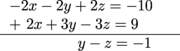Separately, let's multiply Row 1 by -5 and add it to Row 3.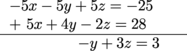Now let's rewrite our set of equations:

x + yz = 5
yz = -1
-y + 3z = 3

If we now add our Row 2 and our Row 3, the y variables will cancel out.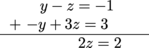Finally, let's write our system of equations in triangular form:

x + yz = 5
yz = – 1
z = 1

Solving this from the bottom up, we will substitute the z = 1 into Row 2, giving us a value of y = 0, and finally both of those values into Row 1 giving x = 6. Our solution is (6, 0, 1).

### Sample Problem

Solve this system of equations using Gaussian elimination.

6x – 2y + z = -30
2x + 3y – 5z = 19
-3x – 4y + 8z = -27

Step 1: Multiply Row 2 by -3, add to Row 1.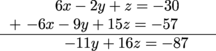Step 2: Multiply Row 3 by 2, add to Row 1.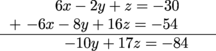Step 3: Rewrite.

6x – 2y + z = -30
-11y + 16z = -87
-10y + 17z = -84

Step 4: Multiply the new Row 2 by -10, multiply the new Row 3 by 11 and add together.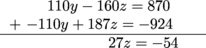Step 5: Rewrite in triangular form and solve backwards.

6x – 2y + z = -30
-11y + 16z = -87
z = -2

So -11y + 16(-2) = -87, y = 5, then 6x – 2(5) + -2 = -30, x = -3. Our solution is (-3, 5, -2).

Step 6: Collapse on the floor and curse Gauss.